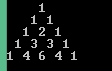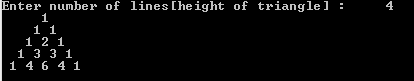## Saturday, 30 January 2016

### Cpp Program to print Pascals triangle

This is a Cpp Program which prints pascals triangle based on the input given.Pascals Triangle

Download Code
PROGRAM :

```#include <iostream>

using namespace std;

int main()
{
int i,j,x,n,s;
cout << "Enter number of lines[height of triangle] : \t";
cin >> n;
for(i=0; i<=n; i++)
{
x=1;
for(s=1; s<=n-i; s++)
cout << " ";
for(j=1; j<=i+1; j++)
{
cout << " " << x;
x=x*(i-j+1)/j;
}
cout << "\n";
}
return 0;
}
```

Download Code
OUTPUT :Cpp Program to print pascals triangle